# Selina Solutions Concise Maths Class 10 Chapter 5 Quadratic Equations Exercise 5(F)

Quadratic equations find themselves applied in various forms. In this exercise, other miscellaneous problems are introduced to the students. Students having any difficulties in solving problems can utilise the Selina Solutions for Class 10 Maths. It is a great asset to students created by subject experts at BYJU’S. The solutions to problems pertaining to this exercise can be found in the Concise Selina Solutions for Class 10 Maths Chapter 5 Quadratic Equations Exercise 5(F) PDF provided below.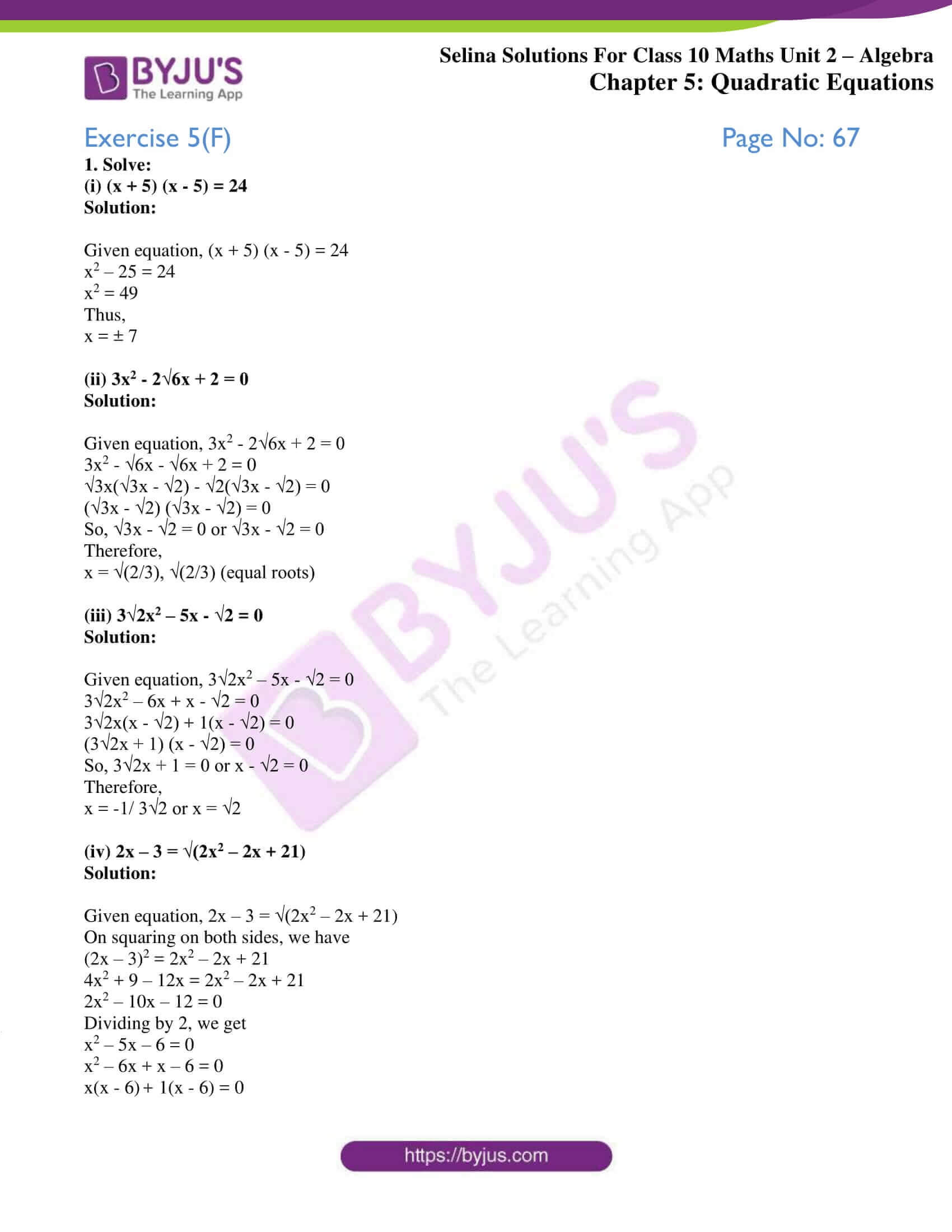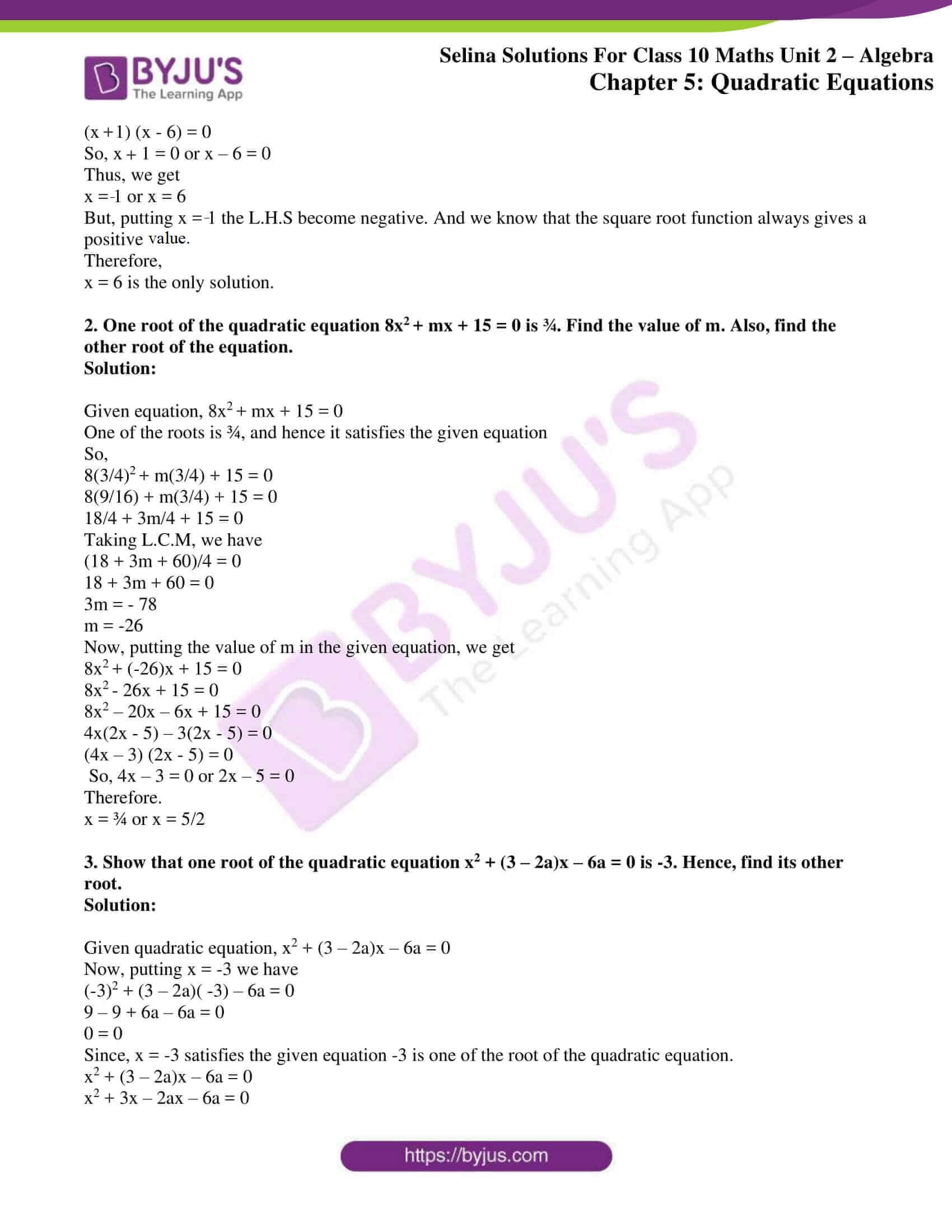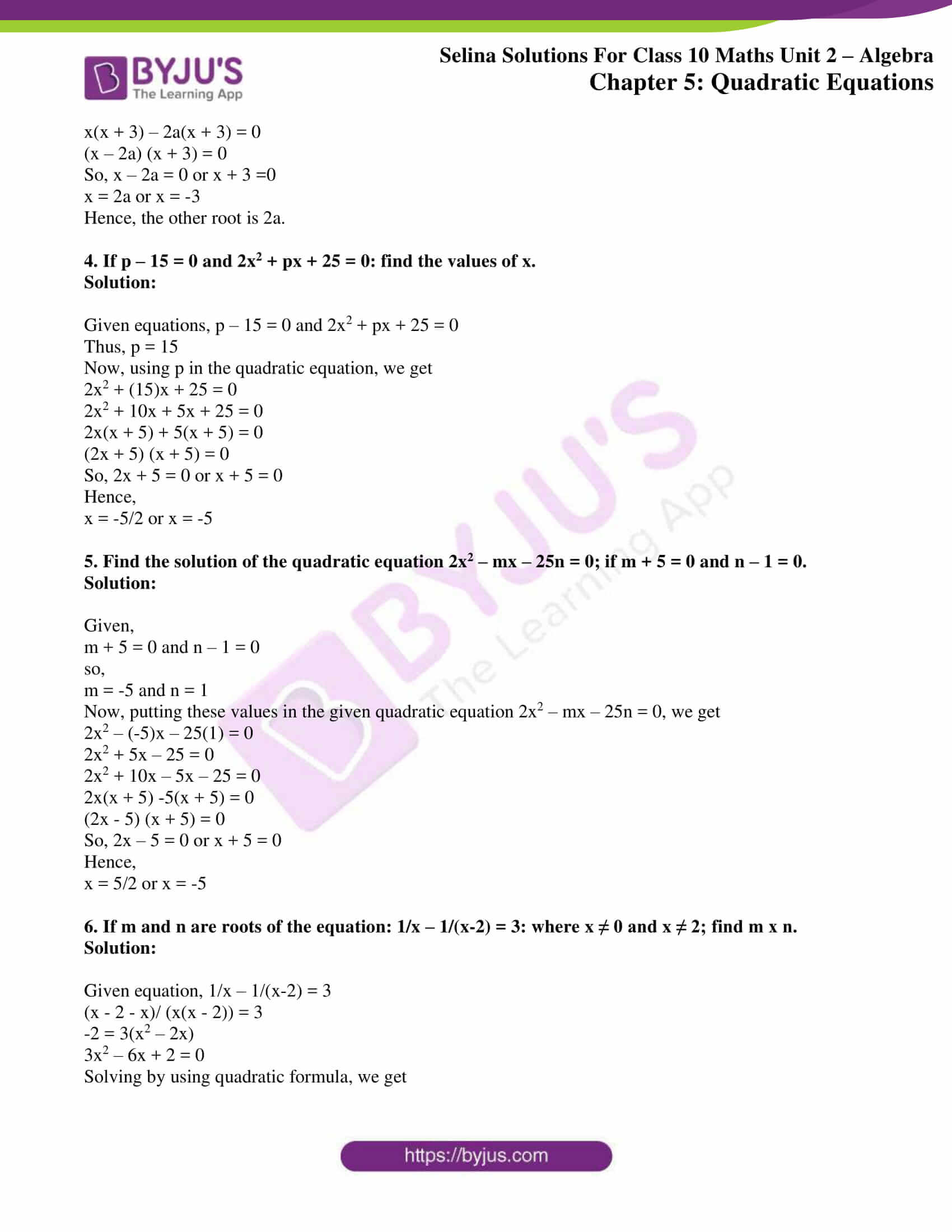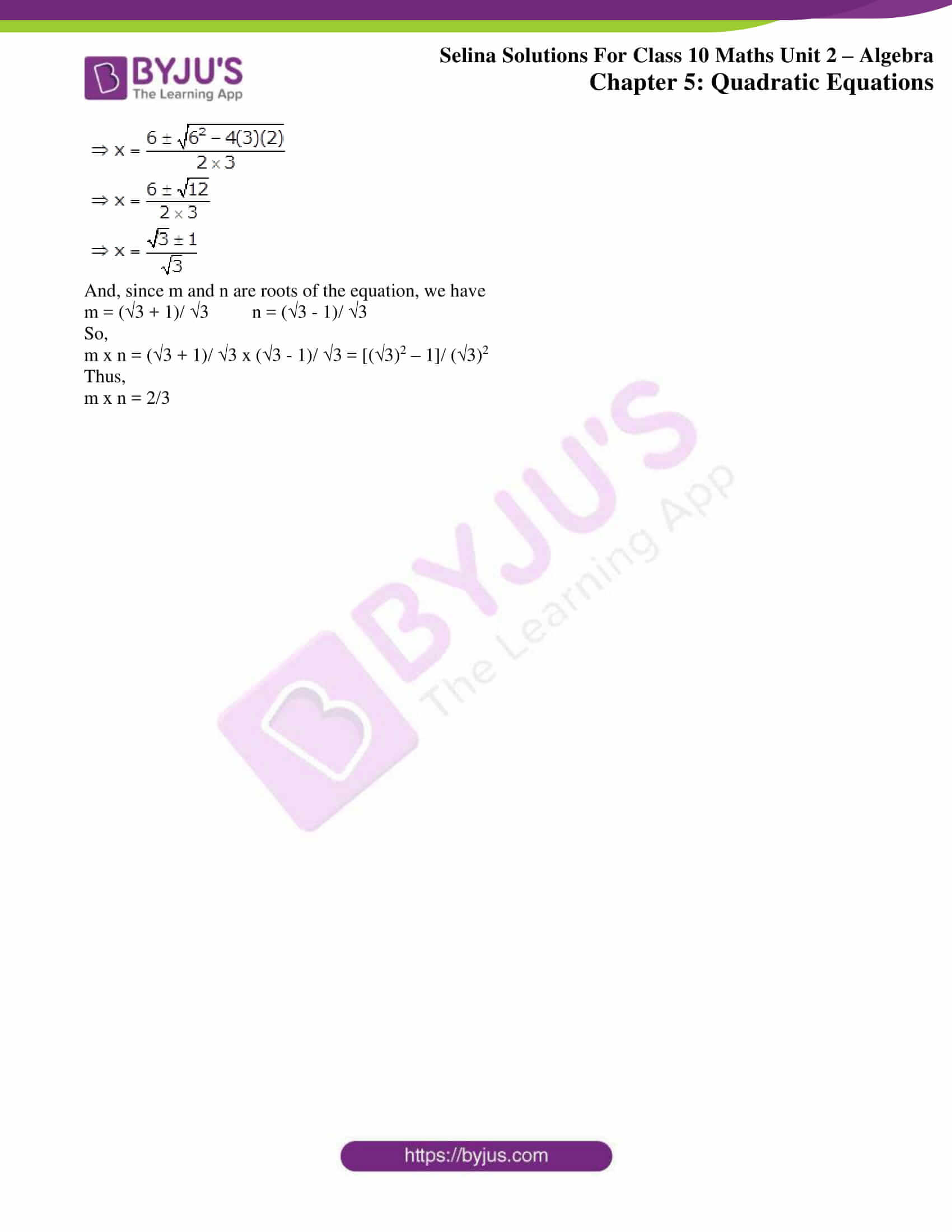### Access other exercise solutions of Class 10 Maths Chapter 5 Quadratic Equations

Exercise 5(A) Solutions

Exercise 5(B) Solutions

Exercise 5(C) Solutions

Exercise 5(D) Solutions

Exercise 5(E) Solutions

### Access Selina Solutions Concise Maths Class 10 Chapter 5 Quadratic Equations Exercise 5(F)

1. Solve:

(i) (x + 5) (x – 5) = 24

Solution:

Given equation, (x + 5) (x – 5) = 24

x2 – 25 = 24

x2 = 49

Thus,

x = ± 7

(ii) 3x2 – 2√6x + 2 = 0

Solution:

Given equation, 3x2 – 2√6x + 2 = 0

3x2 – √6x – √6x + 2 = 0

√3x(√3x – √2) – √2(√3x – √2) = 0

(√3x – √2) (√3x – √2) = 0

So, √3x – √2 = 0 or √3x – √2 = 0

Therefore,

x = √(2/3), √(2/3) (equal roots)

(iii) 3√2x2 – 5x – √2 = 0

Solution:

Given equation, 3√2x2 – 5x – √2 = 0

3√2x2 – 6x + x – √2 = 0

3√2x(x – √2) + 1(x – √2) = 0

(3√2x + 1) (x – √2) = 0

So, 3√2x + 1 = 0 or x – √2 = 0

Therefore,

x = -1/ 3√2 or x = √2

(iv) 2x – 3 = √(2x2 – 2x + 21)

Solution:

Given equation, 2x – 3 = √(2x2 – 2x + 21)

On squaring on both sides, we have

(2x – 3)2 = 2x2 – 2x + 21

4x2 + 9 – 12x = 2x2 – 2x + 21

2x2 – 10x – 12 = 0

Dividing by 2, we get

x2 – 5x – 6 = 0

x2 – 6x + x – 6 = 0

x(x – 6) + 1(x – 6) = 0

(x + 1) (x – 6) = 0

So, x + 1 = 0 or x – 6 = 0

Thus, we get

x = -1 or x = 6

But, putting x = -1 the L.H.S become negative. And we know that the square root function always gives a positive value.

Therefore,

x = 6 is the only solution.

2. One root of the quadratic equation 8x2 + mx + 15 = 0 is ¾. Find the value of m. Also, find the other root of the equation.

Solution:

Given equation, 8x2 + mx + 15 = 0

One of the roots is ¾, and hence it satisfies the given equation

So,

8(3/4)2 + m(3/4) + 15 = 0

8(9/16) + m(3/4) + 15 = 0

18/4 + 3m/4 + 15 = 0

Taking L.C.M, we have

(18 + 3m + 60)/4 = 0

18 + 3m + 60 = 0

3m = – 78

m = -26

Now, putting the value of m in the given equation, we get

8x2 + (-26)x + 15 = 0

8x2 – 26x + 15 = 0

8x2 – 20x – 6x + 15 = 0

4x(2x – 5) – 3(2x – 5) = 0

(4x – 3) (2x – 5) = 0

So, 4x – 3 = 0 or 2x – 5 = 0

Therefore.

x = ¾ or x = 5/2

3. Show that one root of the quadratic equation x2 + (3 – 2a)x – 6a = 0 is -3. Hence, find its other root.

Solution:

Given quadratic equation, x2 + (3 – 2a)x – 6a = 0

Now, putting x = -3 we have

(-3)2 + (3 – 2a)( -3) – 6a = 0

9 – 9 + 6a – 6a = 0

0 = 0

Since, x = -3 satisfies the given equation -3 is one of the root of the quadratic equation.

x2 + (3 – 2a)x – 6a = 0

x2 + 3x – 2ax – 6a = 0

x(x + 3) – 2a(x + 3) = 0

(x – 2a) (x + 3) = 0

So, x – 2a = 0 or x + 3 =0

x = 2a or x = -3

Hence, the other root is 2a.

4. If p – 15 = 0 and 2x2 + px + 25 = 0: find the values of x.

Solution:

Given equations, p – 15 = 0 and 2x2 + px + 25 = 0

Thus, p = 15

Now, using p in the quadratic equation, we get

2x2 + (15)x + 25 = 0

2x2 + 10x + 5x + 25 = 0

2x(x + 5) + 5(x + 5) = 0

(2x + 5) (x + 5) = 0

So, 2x + 5 = 0 or x + 5 = 0

Hence,

x = -5/2 or x = -5

5. Find the solution of the quadratic equation 2x2 – mx – 25n = 0; if m + 5 = 0 and n – 1 = 0.

Solution:

Given,

m + 5 = 0 and n – 1 = 0

so,

m = -5 and n = 1

Now, putting these values in the given quadratic equation 2x2 – mx – 25n = 0, we get

2x2 – (-5)x – 25(1) = 0

2x2 + 5x – 25 = 0

2x2 + 10x – 5x – 25 = 0

2x(x + 5) -5(x + 5) = 0

(2x – 5) (x + 5) = 0

So, 2x – 5 = 0 or x + 5 = 0

Hence,

x = 5/2 or x = -5

6. If m and n are roots of the equation: 1/x – 1/(x-2) = 3: where x ≠ 0 and x ≠ 2; find m x n.

Solution:

Given equation, 1/x – 1/(x-2) = 3

(x – 2 – x)/ (x(x – 2)) = 3

-2 = 3(x2 – 2x)

3x2 – 6x + 2 = 0

Solving by using quadratic formula, we get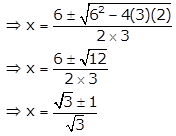And, since m and n are roots of the equation, we have

m = (√3 + 1)/ √3 n = (√3 – 1)/ √3

So,

m x n = (√3 + 1)/ √3 x (√3 – 1)/ √3 = [(√3)2 – 1]/ (√3)2

Thus,

m x n = 2/3